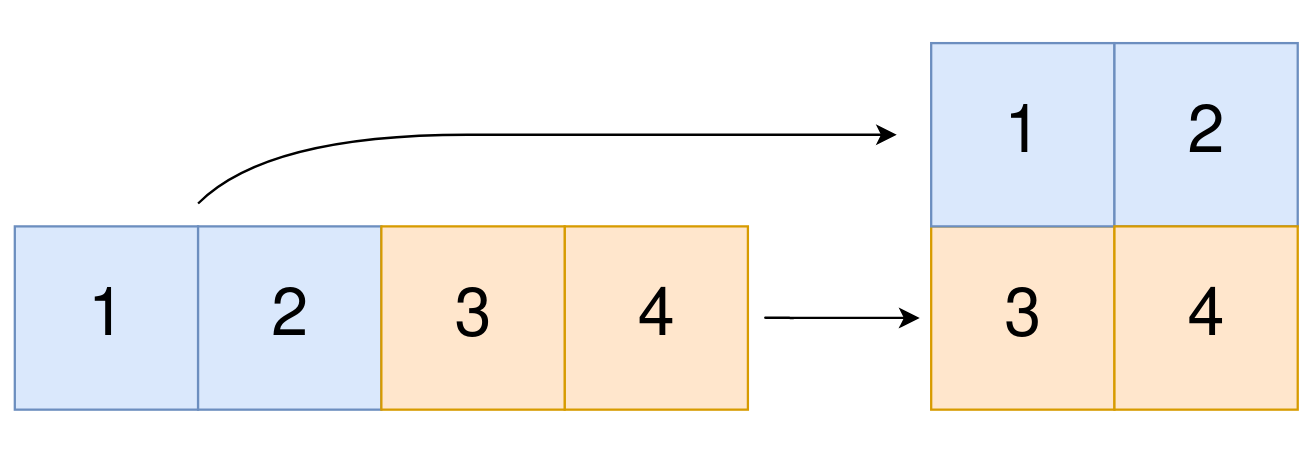# Leetcode 2022 - Convert 1D Array Into 2D Array

Note:

• Know how to convert 2D index into 1D:
• [i, j] => i * n + j. (i*n give us total grids before the curr row then we add j to that).
• i * n + j => [floor(i/n), i %n].

Question:

You are given a 0-indexed 1-dimensional (1D) integer array original, and two integers, m and n. You are tasked with creating a 2-dimensional (2D) array with m rows and n columns using all the elements from original.

The elements from indices 0 to n - 1 (inclusive) of original should form the first row of the constructed 2D array, the elements from indices n to 2 * n - 1 (inclusive) should form the second row of the constructed 2D array, and so on.

Return an m x n 2D array constructed according to the above procedure, or an empty 2D array if it is impossible.

Example:Code: